# The Kopelkides-definition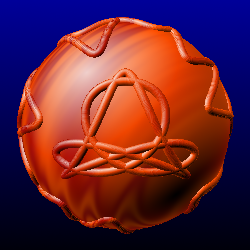Definition:
In a n-dimensional metric room (M, d) are moving time equally n points Pn(t). The path of a point A(t) within this room is a Kopelkide, if the distances dn of this point at each time t to all points Pn(t) are each constant.

The point A is called head-point, the points Pn are the conduct-points, they move upon the conduct-lines.

The Kopelkide is called fixed, if also the distances aij of the conduct-points to one another are syncing constant.

Example of use out of the technique

Pedal modibike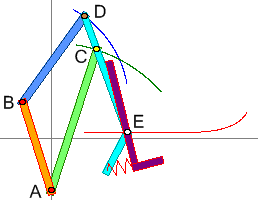In the picture above you see a crane of dispatch converted in a pedal for the Modibike. The yellow conduct-point C moves upon the green circular arc around A, the brown conduct-point D moves upon the blue circular arc around B. The white head-point E has got at any time the same distance towards C and D, it moves upon the red Kopelkide. Because the distance of both conduct-points C and D is always equal, here we have a fixed 2-dimensional Kopelkide of the plane.

Theorem of the Kopelkiden-condition:
The metric room M is complete and steadily infinite standardised and applies Pi(t) ≠ Pj(t) for all t ∈ ℝ, in this case at least one Kopelkide is unique determined, if applies: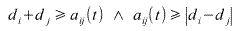Here di means the always constant distance of the head-point A(t) from the conduct-point Pi(t), (i ≤ n) and aij(t) is the distance from the conduct-point Pi(t) towards the conduct-point Pj(t).

Steadily infinite standardised means, there is no upper limit for the distance and there are no gaps in the values range at the definition of the distance. The values of range is W = { [0, ∞] }.

Proof:
Because it is a metric room, the triangle inequality is valid. Here:
a ij(t) ≤ d i + d j
d i ≤ a ij(t) + d j
d j ≤ a ij(t) + d iBy changing the last two equations you get the condition directly.

Is there an upper limit for the distance (like e.g. the digit π as the distance from pole to pole on the unit ball with Euclidean clearance), than the distances of the head-point towards the conduct-points are not allowed to outrun it, because than you could not measure them.

Number of the Kopelkides

About the number of possible Kopelkides you cannot say immediately something. It depends from the room. It is clear, that on a surface of a ball resp. plane (you can see the plane as a ball with radius infinite) is 0, 1, 2 or infinite. The proof takes place algebraic: you put up the formula for the points of intersection of two circles on the surface of a ball. That leads to a square-root equation with 0, 1 or 2 solutions for the case that the middles of the circles are not situated on one and the same original straight line. On the girthed area of a cylinder there can be up to 4 countable points of intersection and therefore the number of Kopelkides is there: {0, 1, 2, 3, 4, ∞}.

On a starfish with 5 beams it can be countable up to 10.

3D-Stereoscope girthed area of a cylinder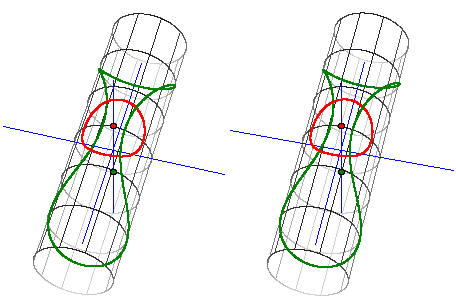Points of the green/red curve have the same distance from the green/red point
4 points of intersection

(to see it in 3D just look with the left/right eye on the right/left picture and bring the pictures to interleave)

Example: discontinuity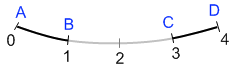a piece of a curve with metric and gap

The picture shows a 1-dimensional room with metric. The points are only allowed, where the curve is black. Between the points B and C is a gap with the length 2. Therefore the values range of the clearance is:
W = {[0, 1] and [2, 4]}.
There are no pairs of points for the distances ]1, 2[ and ]4, ∞[. The three axioms of the metric room are fulfilled despite of the gap. The clearance is not continuously and not infinite.

Theorem:
When the movements of the conduct-points are periodically and stand the cycle duration in a rational ratio to one another, than the Kopelkides are closed, if the theorem of the Kopelkiden-condition is completely complied.

Proof:
If you take without confinement of the universality two cycle duration and divide the lower cycle duration Ti through the higher Tj, than you get a rational digit. This digit is writable as a / b with a, b ∈ ℕ.
If you now run b times the cycle duration, than Pj has run b times its period and Pi conforming b * a / b = a times. a and b are natural numbers. Therefore both points stand at the same time at the same place again. Now you can sum up both cycle duration to a new cycle duration a*Ti = b*Tj and utilize the same procedure with the next cycle duration Tk of the conduct-point Pk until all conduct-points are worked off. Because the di, dj ... are independent of the time, all head-points are at the same place once again. q.e.d.

A 2-dimensional plane Kopelkide
(Double Dog)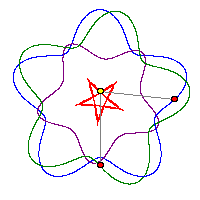master and mistress commute around a circle-curve -
the dog in the middle always holds the same distance to both

The name "Double Dog" comes from the dog-curves (traktrix), which only have one master in 2 dimensions.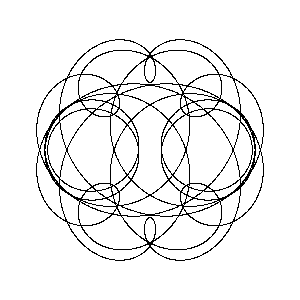another Kopelkide

If you let the curves of master and mistress vary a bit, the Kopelkide varies a bit, too. This leads to beautiful animations like this: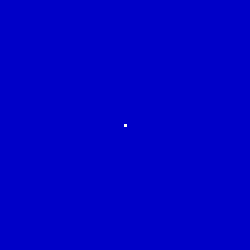lotus

And the best: the richness of forms is infinite and many of them can be tinkered in wood.